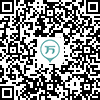142:002019年成人高考（专升本）《大学语文》真题
1～20小题，每小题2分，共40分。在每小题给出的四个选项中，只有一项是符合题目要求的，把所选项前的字母填在题后的括号内。
1
(单项选择题)

• A.

柳永《八声甘州》

• B.

曹操《短歌行》

• C.

屈原《国殇》

• D.

李清照《声声慢》

• A
• B
• C
• D

2
(单项选择题)

• A.

新乐府运动的倡导者

• B.

唐宋八大家之一

• C.

号醉翁，晚年自称六一居士

• D.

著有《新五代史》

• A
• B
• C
• D

3
(单项选择题)

• A.

创造社

• B.

文学研究会

• C.

新月社

• D.

中国左翼作家联盟

• A
• B
• C
• D

4
(单项选择题)

“明月松间照，清泉石上流”使用的修辞方法是（　）。

• A.

排比

• B.

拟人

• C.

夸张

• D.

对偶

• A
• B
• C
• D

5
(单项选择题)

• A.

《陌上桑》

• B.

《行路难》

• C.

《饮酒》

• D.

《杜陵叟》

• A
• B
• C
• D

6
(单项选择题)

• A.

论述了“大同”与“小康”的不同

• B.

为增强说理气势，多处使用夸张、比喻等修辞手法

• C.

间接反映了对现实的不满

• D.

表达了孔子对原始共产主义社会的向往

• A
• B
• C
• D

7
(单项选择题)

• A.

《向太阳》

• B.

《火把》

• C.

《大堰河——我的保姆》

• D.

《北方》

• A
• B
• C
• D

8
(单项选择题)

• A.

欧·亨利

• B.

莫泊桑

• C.

契诃夫

• D.

福楼拜

• A
• B
• C
• D

9
(单项选择题)

《冯谖客孟尝君》采用了欲扬先抑的表现手法，下列不属于贬抑手法的一项是（　）。

• A.

孟尝君曰：“客何好？”曰：“客无好也。”

• B.

左右皆恶之，以为贪而不知足。

• C.

孟尝君不说，曰：“诺。先生休矣！

• D.

孟尝君为相数十年，无纤介之祸者；冯谖之计也。

• A
• B
• C
• D

10
(单项选择题)

• A.

《蚀》《子夜》《林家铺子》《寒夜》都是茅盾的代表作品

• B.

《雷雨日出》《原野》《北京人》都是曹禺的话剧作品

• C.

“爱情三部曲”“农村三部曲”“激流三部曲”的作者是巴金

• D.

郭沫若的历史剧《屈原》《虎符》《蔡文姬》写于抗战时期

• A
• B
• C
• D

11
(单项选择题)

• A.

郭沫若

• B.

徐志摩

• C.

闻一多

• D.

艾青

• A
• B
• C
• D

12
(单项选择题)

• A.

“东面而视，不见水端”，描写了北海的辽阔

• B.

“两涘渚崖之间，不辨牛马”，描写了黄河水上涨的景色

• C.

“吾长见笑于大方之家”，说明河伯认识到了自己的局限

• D.

“天下之水，莫大于海”，写出了北海若骄傲自得的心态

• A
• B
• C
• D

13
(单项选择题)

• A.

郭橐驼对这个绰号很反感

• B.

郭橐驼懂得顺应树木生长的规律

• C.

郭橐驼移栽树木时会换上新土

• D.

郭橐驼早晚都会去照料树木

• A
• B
• C
• D

14
(单项选择题)

• A.

议论文

• B.

说明文

• C.

记叙文

• D.

散文

• A
• B
• C
• D

15
(单项选择题)

• A.

比喻

• B.

用典

• C.

拟人

• D.

叠字

• A
• B
• C
• D

16
(单项选择题)

• A.

夫祸患常积于忽微，而智勇多困【于】所溺

• B.

王知如此，则无望民之多【于】邻国也

• C.

此其自多也，不似尔向之自多【于】水乎

• D.

邦分崩离析而不能守也，而谋动干戈【于】邦内

• A
• B
• C
• D

17
(单项选择题)

• A.

生庄公【及】共叔段

公曰：“无庸，将自【及】。”

• B.

秋水【时】至，百川灌河

其先曰李信，秦【时】为将

• C.

大军不知广所【之】，故弗从

观者见其然，从而尤【之】

• D.

橐驼非能使木寿【且】也

昔者先王以为东蒙主，【且】在邦城之中矣

• A
• B
• C
• D

18
(单项选择题)

• A.

当【是】时，弃城而图存者，不可一二数

• B.

【是】以泰山不让土壤，故能成其大

• C.

雁过也，正伤心，却【是】旧时相识

• D.

夫如【是】，故远人不服，则修文德以来之

• A
• B
• C
• D

19
(单项选择题)

• A.

【将】子无怒，秋以为期　将：将要

• B.

【契阔】谈宴，心念旧恩　契阔：聚散

• C.

女也不【爽】，士贰其行　爽：愉快、快乐

• D.

守着窗儿，独自【怎生】得黑　怎生：怎么生活

• A
• B
• C
• D

20
(单项选择题)

• A.

闻道百，【以为莫己若者】，我之谓也

以为莫己若者：认为没有谁如同自己一样

• B.

今乃弃黔首以资敌国，【却宾客以业诸侯】

却宾客以业诸侯：驱逐宾客使诸侯威就功业

• C.

填然鼓之，兵刃既接，【弃甲曳兵而走】

弃甲曳兵而走：抛弃了铠甲、拖着士兵离开了

• D.

【制，岩邑也】，叔死焉，佗邑为命

制，岩邑也；制是险要的城邑

• A
• B
• C
• D